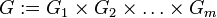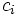# Orthogonal direct sum of cocycles across acting groups

## Definition

Suppose$m$ and$n$ are natural numbers. Consider a bunch of groups$G_1, G_2, \dots, G_m$, all equipped with actions on an abelian group$A$. Suppose, for each$i$, that we have a$n$-cocycle$c_i: G_i^n \to A$ for the action of$G_i$ on$A$.

Then, consider$G := G_1 \times G_2 \times \dots \times G_m$. If all the$G_i$-actions on$A$ commute with each other, then we get an induced$G$-action on$A$. We can define a$n$-cocycle$c$ for this action in terms of$c_i$s, as the orthogonal direct sum of cocycles as follows:

First, view$G$ as an internal direct product, so each$G_i$ is identified with a subgroup of$G$. Now, define:$c((g_{11},g_{12},\dots, g_{1m}),(g_{21},g_{22},\dots,g_{2m}), \dots,(g_{n1},g_{n2},\dots,g_{nm}) = \sum_{i=1}^m c_i(g_{1i},g_{2i},\dots,g_{ni})$

where the summation on the right happens in$A$.

This does indeed give a cocycle.